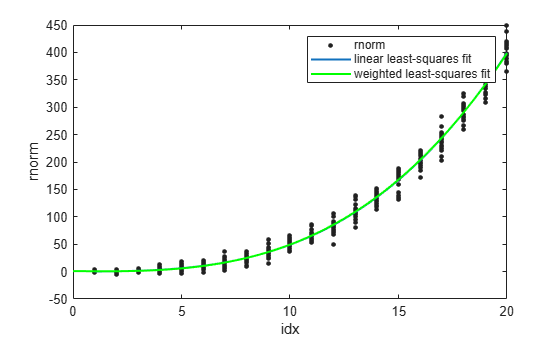# Improve Model Fit with Weights

This example shows how to fit a polynomial model to data using both the linear least-squares method and the weighted least-squares method for comparison.

Generate sample data from different normal distributions by using the `randn` function.

```rng("default") % For reproducibility rnorm = []; idx = []; for k=1:20 r = k*randn([20,1]) + (1/20)*(k^3); rnorm = [rnorm;r]; idx = [idx;ones(20,1).*k]; end ```

The dependent variable `rnorm` contains sample data from 20 normal distributions. The independent variable `idx` contains integers indicating whether two elements in `rnorm` are sampled from the same normal distribution.

Fit a third-degree polynomial model to `idx` and `rnorm`. Return information about the coefficient estimates and the algorithm used to fit the model.

`[p3fit,~,fitinfo] = fit(idx,rnorm,"poly3")`
```p3fit = Linear model Poly3: p3fit(x) = p1*x^3 + p2*x^2 + p3*x + p4 Coefficients (with 95% confidence bounds): p1 = 0.05156 (0.0438, 0.05932) p2 = -0.03993 (-0.2875, 0.2076) p3 = 0.1418 (-2.124, 2.408) p4 = 0.0462 (-5.585, 5.678) ```
```fitinfo = struct with fields: numobs: 400 numparam: 4 residuals: [400×1 double] Jacobian: [400×4 double] exitflag: 1 algorithm: 'QR factorization and solve' iterations: 1 ```

`p3fit` contains the estimates for the model coefficients with 95% confidence intervals. The default fitting method for fitting a polynomial model is linear least squares. `fitinfo` contains information about the fitting algorithm used to fit the coefficients to the data. The error in the data can be estimated by the residuals stored in `fitinfo`.

Plot the residuals using a stem plot.

```stem(idx,fitinfo.residuals) xlabel("idx") ylabel("residuals")```The plot of the residuals shows that their variance increases as the values in `idx` increase. The nonconstant variances across the different values of `idx` indicate that the weighted least-squares fitting method is more appropriate for calculating the model coefficients.

Create a vector of zeros for later storage of the weights.

`W = zeros(length(rnorm),1);`

The weights you supply transform the residual variances so that they are constant for different values of `idx`. Define the weight for each element in `rnorm` as the reciprocal of the residual variance for the corresponding value in `idx`. Then fit the model with the weights.

```for k=1:20 rnorm_idx = rnorm(idx==k); recipvar = 1/var(rnorm_idx); w = (idx==k).*recipvar; W = W+w; end [wp3fit,~,wfitinfo] = fit(idx,rnorm,"poly3","Weights",W)```
```wp3fit = Linear model Poly3: wp3fit(x) = p1*x^3 + p2*x^2 + p3*x + p4 Coefficients (with 95% confidence bounds): p1 = 0.04894 (0.04419, 0.0537) p2 = 0.03601 (-0.08744, 0.1595) p3 = -0.4262 (-1.253, 0.4009) p4 = 0.9836 (-0.1959, 2.163) ```
```wfitinfo = struct with fields: numobs: 400 numparam: 4 residuals: [400×1 double] Jacobian: [400×4 double] exitflag: 1 algorithm: 'QR factorization and solve' iterations: 1 ```

`wp3fit` and `wfitinfo` contain the results of the weighted least-squares fitting.

Display `p3fit`, `wp3fit`, and `rnorm` in the same plot.

```plot(p3fit,idx,rnorm) hold on plot(wp3fit,"g") xlabel("idx") ylabel("rnorm") legend(["rnorm","linear least-squares fit","weighted least-squares fit"]) hold off```The plot shows `wp3fit` closely tracking `p3fit`.

You can determine whether `wp3fit` is a better fit than `p3fit` by plotting the residuals.

```stem(idx,wfitinfo.residuals) xlabel("idx") ylabel("residuals")```The output shows that the `wp3fit` residuals are smaller than the `p3fit` residuals. The variances of the `wp3fit` residuals are also more similar for different values of `idx` than the variances of the `p3fit` residuals.